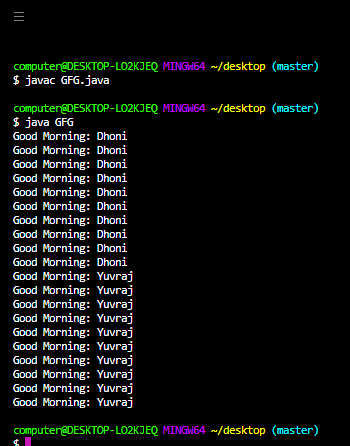Open in App
Not now

# Object Level Lock vs Class Level Lock in Java

• Difficulty Level : Basic
• Last Updated : 19 Apr, 2022

Synchronized is the modifier applicable only for methods and blocks but not for classes and variables. If multiple threads are trying to operate simultaneously on the same java object then there may be a chance of data inconsistency problem to overcome this problem we should go for a ‘synchronized‘ keyword. If a method or block declares as synchronized then at a time only one thread is allowed to execute that method or block on the given object so that the data inconsistency problem will be resolved. Internally synchronization concept is implemented by using the lock. Every object in java has a unique lock. Whenever we are using a ‘synchronized‘ keyword then only the lock concept will come into the picture.

If a thread want’s to execute a synchronized method on the given object first it has to get a lock of that object once the thread got the lock then it is allowed to execute any synchronized method on that object. Acquiring and releasing lock internally takes care of by JVM and the programmer is not responsible for this activity. While a thread executing a synchronized method on the given object the remaining threads are not allowed to execute any synchronized method simultaneously on the same object. But remaining threads are allowed to execute non-synchronized methods simultaneously.

Object Level Lock is a mechanism where every object in java has a unique lock which is nothing but an objectlevel lock. If a thread wants to execute a synchronized method on the given object then the thread first required the object level lock once the thread gets object level lock then it is allowed to execute any synchronized method on the given object and once the method execution completed automatically thread releases the lock of that object.

Example:

## Java

 `// Java program to illustrate Object Level Lock Concept` `// Import required packages``import` `java.io.*;``import` `java.util.*;` `// Class 1``// Helper Class 1``// Consists of synchronized method wish``class` `Display {` `    ``// Declaring Non-static wish method``    ``public` `void` `wish(String name)``    ``{` `        ``// synchronizing wish method``        ``// and getting the lock of current object``        ``synchronized` `(``this``)``        ``{` `            ``for` `(``int` `i = ``1``; i <= ``10``; i++) {` `                ``// display message only``                ``System.out.print(``"Good Morning: "``);` `                ``// Try block to check. for exceptions``                ``try` `{` `                    ``// Putting thread on sleep for specified``                    ``// time``                    ``// using the sleep() method``                    ``Thread.sleep(``1000``);``                ``}` `                ``// Catch block to handle the exceptions``                ``catch` `(InterruptedException e) {` `                    ``// Print the occurred exception/s``                    ``System.out.println(e);``                ``}``                ``// Display message only``                ``System.out.println(name);``            ``}``        ``}``    ``}``}` `// Class 2``// Helper Class 2 (extending main Thread Class)``// myThread with override the run method and``// consists of parameterized constructor``class` `myThread ``extends` `Thread {``    ``// member variable of this class``    ``Display d;``    ``String name;` `    ``// Constructor(Parameterized) of this class``    ``myThread(Display d, String name)``    ``{` `        ``// this keyword refers to current object itself``        ``this``.d = d;``        ``this``.name = name;``    ``}` `    ``// run() method for thread``    ``public` `void` `run()``    ``{` `        ``// Calling wish method of display class``        ``d.wish(name);``    ``}``}` `// Class 3``// Main Class``class` `GFG {` `    ``// Main driver method``    ``public` `static` `void` `main(String[] args)``    ``{` `        ``// Creating display class(Class 1) object``        ``// in the main() method``        ``Display d = ``new` `Display();` `        ``// Creating thread objects in main method()``        ``myThread t1 = ``new` `myThread(d, ``"Dhoni"``);``        ``myThread t2 = ``new` `myThread(d, ``"Yuvraj"``);` `        ``// Starting the threads using the start() method``        ``t1.start();``        ``t2.start();``    ``}``}`Class Level Lock is a mechanism where every class in java has a unique lock which is nothing but a class level lock. If a thread wants to execute a static synchronized method then the thread requires a class level lock once the thread gets a class level lock then it is allowed to execute any static synchronized method of that class. Once method execution completes automatically thread releases the lock. While a thread executing a static synchronized method the remaining thread is not allowed to execute any static synchronized method of that class simultaneously.

Example:

## Java

 `// java program to illustrate Class Level Lock Concept` `// Importing required packages``import` `java.io.*;``import` `java.util.*;` `// Class 1``// Helper Class``// Consist of synchronized method wish``class` `Display {` `    ``// Declaring static wish method``    ``public` `static` `void` `wish(String name)``    ``{` `        ``// synchronizing wish method``        ``// and getting the lock of display class``        ``synchronized` `(Display.``class``)``        ``{` `            ``for` `(``int` `i = ``1``; i <= ``10``; i++) {` `                ``// Display message only``                ``System.out.print(``"Good Morning: "``);` `                ``// Try block to check for exceptions``                ``try` `{``                    ``// Putting thread on sleep for specified``                    ``// time``                    ``//  using the sleep() method``                    ``Thread.sleep(``1000``);``                ``}` `                ``// Catch block to handle the exception``                ``catch` `(InterruptedException e) {` `                    ``// Throwing exception``                    ``System.out.println(e);``                ``}``                ``// Display message``                ``System.out.println(name);``            ``}``        ``}``    ``}``}` `// C;asss 2``// Helper Class (extends the Thread class)``// myThread with override the run method``// as per our requirements it also consists``// of parameterized constructor``class` `myThread ``extends` `Thread {``    ``// Member variables of this class``    ``Display d;``    ``String name;` `    ``// Constructor of this class``    ``myThread(Display d, String name)``    ``{` `        ``// This keyword refers to current object itself``        ``this``.d = d;``        ``this``.name = name;``    ``}` `    ``// run method for thread/s``    ``public` `void` `run()``    ``{` `        ``// Calling wish method of display class``        ``d.wish(name);``    ``}``}` `// Class 3``// Main Class``class` `GFG {` `    ``// Main driver method``    ``public` `static` `void` `main(String[] args)``    ``{` `        ``// Creating Display class(Class 1) object``        ``// in the main() method``        ``Display d = ``new` `Display();` `        ``// Creating a thread objects``        ``myThread t1 = ``new` `myThread(d, ``"Dhoni"``);``        ``myThread t2 = ``new` `myThread(d, ``"Yuvraj"``);` `        ``// Starting the threads using start() method``        ``t1.start();``        ``t2.start();``    ``}``}`My Personal Notes arrow_drop_up